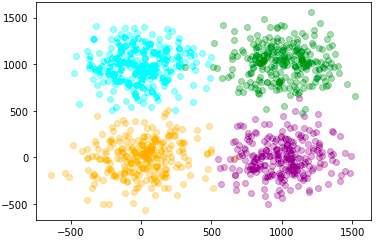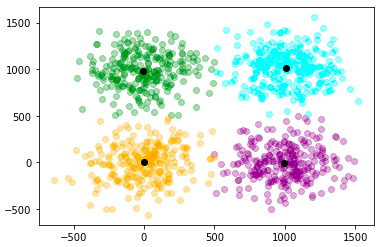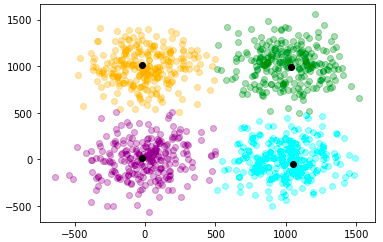# Mini Batch KMeans

## Introduction

Before going onto the main topic lets us briefly discuss clustering and K-means clustering.

### Clustering

Clustering is an unsupervised learning approach since there is no ground truth to compare the clustering algorithm's output to the true labels to evaluate its success. We just want to look at the data's structure by dividing the data points into various subgroups. In other words, clustering is a task of identifying subgroups in the data such that data points in the same subgroup show some similarities.

### K-means clustering

The Kmeans method is an iterative technique that attempts to split a dataset into K separate non-overlapping subgroups (clusters), where each data point can belong to only one group. It attempts to make intra-cluster data points as similar as feasible while maintaining clusters as distinct (far) as possible. It distributes data points to clusters in such a way that the sum of the squared distances between them and the cluster's centroid (arithmetic mean of all the data points in that cluster) is as little as possible. The less the variance in the clusters, the more homogenous (similar) the data points are.

## Mini Batch KMeans

The primary idea behind the Mini Batch K-means method is to create small random batches of data with a predetermined size so that they may be kept in memory. Each iteration obtains a fresh random sample from the dataset and uses it to update the clusters, and the process is continued until convergence. Each mini-batch updates the clusters using a convex mixture of the prototype values and the data, with a decreasing learning rate as the number of iterations increases. The inverse of the number of data assigned to a cluster during the procedure is this learning rate. Because the influence of incoming data diminishes as the number of iterations grows, convergence can be observed when no changes in the clusters occur for multiple iterations in a row.

### Properties of KMeans

• No need to store the whole dataset in the memory
• At each iteration, the distance between the mini-batch and the k centroids needs to be calculated.
• At each iteration, the user needs to store k centroids and a subset of data in the memory.

Let us implement a small model to check the time difference between K-means and mini-batch K-means.

## Implementation

### Importing Libraries

We have imported MiniBatchKMeans and KMeans models from the sklearn library. To make a dataset we are using the make_blobs function, and we will use matplotlib to plot the dataset. We will use the timeit library to measure the amount of time taken by each model to get trained.

``````from sklearn.cluster import MiniBatchKMeans, KMeans
from sklearn.datasets import make_blobs as mb
import matplotlib.pyplot as plt
import timeit as tm``````

### Preparing the Dataset

Before creating the dataset we will define the centers for the clusters.

Creating a dataset having 1000 data points. The data points are divided into four clusters. The standard deviation around clusters is 200.

``````centre = [[0, 0],
[1000, 0],
[1000, 1000],
[0, 1000]]
dataset, labels = mb(n_samples = 1000, centers = centre, cluster_std = 200)``````

### Plotting the Dataset

Let us plot the dataset that we have created.

``````color = ['orange', 'purple', 'green', 'cyan']
for i in range(len(dataset)):
plt.scatter(dataset[i], dataset[i],
color = color[labels[i]], alpha = 0.3)``````### Training a Model Using K-means Algorithm

Training the model using the dataset we prepared and measuring the amount of time required to train the model.

``````kmeans = KMeans(n_clusters=4, random_state=0)
start = tm.default_timer()
kmeans.fit(dataset)
end = tm.default_timer()
a = kmeans.labels_
centers = kmeans.cluster_centers_
print(end-start)``````

Time taken to train the model:

``0.08108869799980312``

Let us plot the predictions of the model.

``````for i in range(len(dataset)):
plt.scatter(dataset[i],dataset[i], color = color[a[i]], alpha = 0.3)
for i in range(len(centers)):
plt.scatter(centers[i], centers[i], color = 'black')``````As we can see that the clusters are formed with good accuracy. The black dots denote the centroid of the four clusters.

### Training a Model Using Mini Batch K-means Algorithm

Let us train the model using a mini-batch k-means algorithm with a batch size of 20. Passing the same data through the model that we used for the K-means algorithm.

``````kmeans = MiniBatchKMeans(n_clusters=4, batch_size = 20, random_state=0)
start = tm.default_timer()
kmeans.fit(dataset)
end = tm.default_timer()
a = kmeans.labels_
centers = kmeans.cluster_centers_
print(end-start)``````

Time taken to train the model:

``0.039445771999908175``

Let us plot the predictions of the model.

``````for i in range(len(dataset)):
plt.scatter(dataset[i],dataset[i], color = color[a[i]], alpha = 0.3)
for i in range(len(centers)):
plt.scatter(centers[i], centers[i], color = 'black')``````As we can see that predictions of the kmeans and mini-batch kmeans algorithm are nearly equal. But the major difference is the amount of time taken by the model to train. For a dataset of 1000 data points, the kmeans algorithm has taken 0.081 seconds, whereas the mini-batch kmeans algorithm has taken 0.039 seconds.

So making small batches of the dataset and training the model using the small subsets of the dataset is quite efficient.

## FAQs

1. What is Mini Batch KMeans?

A: The Mini-batch K-means clustering algorithm is a variant of the traditional K-means algorithm. It stores data in memory in short, random, fixed-size batches, and then a random sample of the data is gathered and utilized to update the clusters with each iteration.

2. What is the elbow method in KMeans?

A: The elbow approach performs k-means clustering on the dataset for a range of k values (say, 1-10) and then computes an average score for all clusters for each value of k. The distortion score, which is the sum of square distances from each point to its assigned center, is computed by default.

3. What is distortion in Kmeans?

A: "Distortion," according to kmeans, is "the total of the squared deviations between the observations and the associated centroid." It is implemented as the sum of (unsquared) differences.

## Key Takeaways